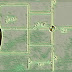More examples..........!!!!

DISCRIPTION
HARSH.MPF - Main program name .
N20-   Referance point command where X0 and Z0 .
N30-   Tool change command select tool no 6 and tool offset 1 .
N40-   Spindle start clockwise at speed 1000 rpm .
N50-   Coolant ON clockwise .
N60-   Rapid command where X0 and Z0 .
N70-   Linear interpolation where X29 and Z-20 ;
N80-   Rapid command where  X32 and Z2 ;
N90-   Linear interpolation where X 28 and Z-20;
N100- Rapid command where X32 and Z2 ;
N110- Linear interpolation  where X27 and Z-20 ;
N120- Rapid command where X32 and Z2 ;
N130- Linear interpolation where X26 and Z-20 ;
N140- Rapid command where X32 and Z2 ;
N150- Linear interpolation where X25 and Z-20 ;
N160- Rapid command where X32 and Z2 ;
N170- Referance point command where X0 and Z0 .
N190- Coolant off.
N200- Main program end.
CNC STEP TURNING PROGRAM FOR FANUCReviewed by harshal on March 14, 2018 Rating: 5